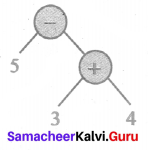Tamilnadu Samacheer Kalvi 6th Maths Solutions Term 2 Chapter 5 Information Processing Ex 5.2

Miscellaneous Practice Problems

Question 1.
Write the missing numbers.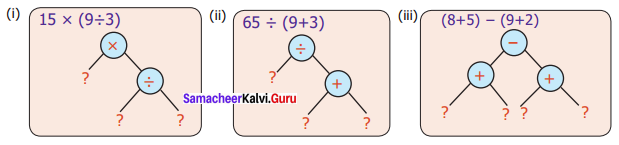Solution:
(i) 15 × (9 ÷ 3)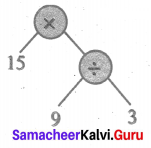(ii) 65 ÷ (9 + 3)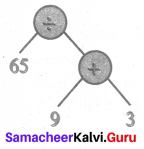(iii) (8 + 5) – (9 + 2)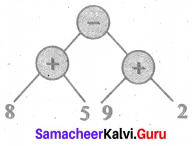Question 2.
Write the missing operations in the trees.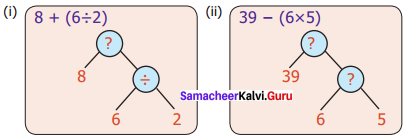Solution:
(i) 8 + (6 ÷ 2)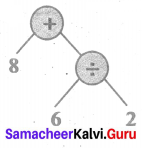(ii) 39 – (6 × 5)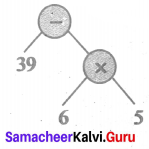Question 3.
Check whether the Tree diagrams are equal or not.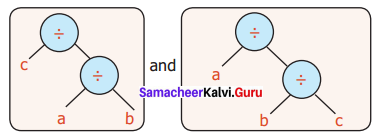Solution:
c ÷ (a ÷ b) ≠ a(b ÷ c)

Challenge Problems

Question 4.
Convert the following questions into tree diagrams:
(i) The number of people who visited a library in the last 5 months was 1210, 2100, 2550, 3160 and 3310. Draw the tree diagram of the total number of people who had used the library for the 5 months.
(ii) Ram had a bank deposit of ₹ 7,55,250 and he had withdrawn ₹ 5,34,500 for educational purpose. Find the amount left in his account. Draw a tree diagram for this.
(iii) In a cycle factory, 1,600 bicycles were manufactured on a day. Draw tree diagram to find the number of bicycles produced in 20 days.
(iv) A company with 30 employees decided to distribute ₹ 90,000 as a special bonus equally among its employees. Draw tree diagram to show how much will each receive?
Solution:
(i) People who visited the library for the past 5 months = 1210 + 2100 + 2550 + 3160 + 3310 = 12,330
Total number of people visited = 12,330
Tree Diagram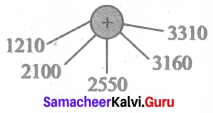(ii) Total bank deposit of Ram = ₹ 7,55,250
Amount withdrawn for educational purpose = ₹ 5,34,500
Amount left in his account = ₹ 2,20,750
Amount left in Ram’s Account = ₹ 2,20,750
Tree Diagram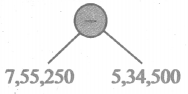(iii) Number of bicycles manufactured in a day = 1600
Number of bicycles manufactured in 20 days = 1600 × 20 = 32,000
Number of bicycles manufactured in 20 days = 32,000
Tree Diagram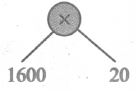(iv) Total amount distributed to 30 employees = 90,000
Amount received by one employee = $$\frac { 90000 }{ 30 }$$ = 3000
Tree Diagram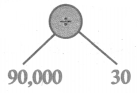Question 5.
Write the numerical expression which gives the answer 10 and also convert into a tree diagram.
Solution:
Numerical expression 15 – (3 + 2)
Tree Diagram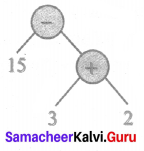Question 6.
Use brackets inappropriate place to the expression 3 × 8 – 5 which gives 19 and convert it into tree diagram for it.
Solution:
Numerical expression (3 × 8) – 5
Tree Diagram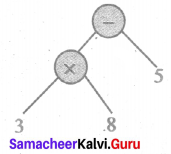Question 7.
A football team gains 3 and 4 points for successive 2 days and loses 5 points on the third day. Find the total points scored by the team and also represent this in the tree diagram.
Solution:
Total points scored by the team = 3 + 4 – 5 = 2
Tree Diagram